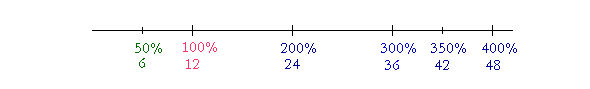S k i l l
i n
A R I T H M E T I C

Lesson 27

# PERCENTS ARE RATIOS

The very first Lesson on Percent is Lesson 3.

Now every statement of percent can be expressed verbally in this way:

"One number is some percent of another number."

For example,

8 is 50% of 16.

Every statement of percent therefore involves three numbers. 8 is called the Amount. 50% is the Percent. 16 is called the Base. The Base always follows "of."

This Lesson is a review of ratio in the language of percent.

 1. How do percents express ratios? Whatever ratio the percent has to 100 percent, that is the ratio we mean. For, per cent is how many for each 100.

What ratio does each of the following express?

50%

Half.  Because 50 is half of 100.

25%

A quarter, or a fourth.  Because 25 is a quarter of 100.

 33 13 %
 A third.   Because if we divide 100 by 3, we get 33 13 .  (Lesson 15.)
 33 13 is a third of 100.

200%

Two times, or twice as much.  Because 200 is two times 100.

250%

1000%

Ten times.   Because 1000 is ten times 100.

Whatever ratio the percent has to 100 percent, that is the ratio we mean.

The Amount

Example 1.   How much is 100% of 12?

Answer.  12.  100% is the whole thing.

Example 2.   How much is 200% of 12?

Answer.  24.  200% is twice as much as 100%.

Example 3.   How much is 300% of 12?

Answer.  36.  300% is three times 100%.

Example 4.   How much is 50% of 12?

Answer.  Translate immediately into the language of ratio:  "How much is half of 12?"  Answer:  6.

 Example 5.    How much is 33 13 % of 12?

Translate:  "How much is a third of 12?"  A third of 12 is 4.

Example 6.   How much is 350% of 12?

Translate:  "How much is a three and a half times 12?"  (350% = 300% + 50%:  Three times plus half.)

Three times 12 is 36.  Half of 12 is 6.  36 + 6 = 42.

We see that more than 100% of 12 is more than 12,while less than 100% will be less.

Example 7.   How much is 1% of \$512?

\$512 ÷ 100 = \$5.12.

Example 8.   How much is 2% of \$512?

Answer.  2% is twice as much as 1%.  1% of \$512 is \$5.12.  Therefore

2%  is  2 × \$5.12 = \$10.24.

Example 9.   How much is 10% of \$434?

Translate:  "How much is a a tenth of \$475?"  (10% means a tenth, because 10 is a tenth of 100.)  To find a tenth of a number, divide it by 10.  To divide a whole number by 10, separate one decimal place:

\$434 ÷ 10 = \$43.4

Money however is written with two decimal places.  Therefore report the answer as

\$43.40.

Example 10.   How much is 20% of \$142?

Answer.   20% is twice as much as 10%.  10% is \$14.20.  Therefore 20% is \$28.40.

The Base

Example 11.   7 is 25% of _?_

Answer.  The Base -- the number that follows of -- is missing.
Translate:

"7 is a quarter of what number?"

7 is a quarter of 28.

Example 12.   10 is 20% of _?_

Translate:  "10 is the fifth part of what number?"  (20% means the fifth part, because 20 is the fifth part of 100.)

10 is the fifth part of 50.

Example 13.   15 is 300% of _?_

Translate:  "15 is the three times what number?"

15 is three times 5.

Example 14.   280 is 1000% of _?_

Translate:  "280 is the ten times what number?"

280 is ten times 28.

The Percent

Example 15.   48 is what percent of 48?

Answer.   100%.  100% is the whole thing!

Example 16.   9 is what percent of 36?

9 is what percent of 36?

9 has what ratio to 36?

Percents are ratios.

Now,

9 is a fourth of 36.

Therefore,

9 is 25% of 36.

25% means a fourth, or a quarter.

Example 17.   35 is what percent of 7?

Translate:   "35 has what ratio to 7?"

35 is five times 7.

The percent, then, will be five times 100:

35 is 500% of 7.

In every case,

The percent has that same ratio to 100.

Example 18.   \$2.50 is what percent of \$250?

Answer.  "\$2.50 is which part of \$250?"

The digits are the same:  2, 5, 0.  But \$2.50 has two decimal places.  It is \$250 divided by 100:

2.50 = 250 ÷ 100.

Therefore,

\$2.50 is 1% of \$250.

(Example 7 above.)

At this point, please "turn" the page and do some Problems.

or

Continue on to the next Section.

www.proyectosalonhogar.com